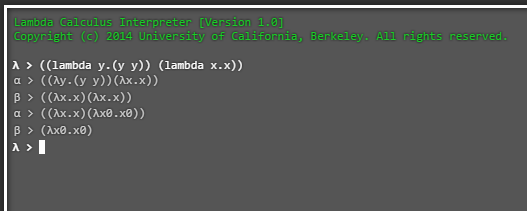# Friday April 14th

## Today I learned:

• Rewatched Dustin Mulcahey’s talk on “Lambda Calculus” from LispNYC group. Also found a neat online Lambda Calculator, but I have to learn the syntax :) —> lambda calculator

## Beta-Reduction## Today I learned…cont’d:

• Watched a great documentary on Titan and Cassini, and some on Vesper. Learned about cryo-volcanoes. I guess this is what happens when you hang out with rocket scientists the evening before. :)

• Learned about Porkchop plots, Delta-v, the Oberth Effect and the Tsiolkovsky rocket equation for calculating orbital maneuver.

• Manacher’s Algorithm -> Palindrome substrings

## Random Kata:

• If you fold a piece of paper that has a thickness of .0001m, calculate how many times you have to fold the paper to make the paper reach the distance of the Earth to the moon.
``````function foldTo(distance) {

var t = .0001; // thickness of paper
var a = distance / t // total length -> distance / thickness => 3840000000000

// check to make sure length or distance is a number above 0

if (distance <= 0){
return null
}

else{
// find the value where 2^n is less than total length, a
for (var i = 1; i < a; i++){
if (Math.pow(2, i) > a){
var a = i;
}
}
}
return parseInt(a) // returns 42

}
``````

## Things to get done:

• C++ quiz will get done, and hopefully posting to C++ discussion board.

• Equivalence lecture and quiz.

## Notes on Equivalence:

Let E1, E2 and E3 be any predicates whatsoever.

## Commutativity

• conjunction: (E1 AND E2) <=> (E2 AND E1). This is self-evident.

• disjunction: (E1 OR E2) <=> (E2 OR E1). This is self-evident.

• equivalence: (E1 <=> E2) <=> (E2 <=> E1). This is self-evident.

## Associativity

In associativity, grouping is not important, so we can ignore the parentheses.

• conjunction: E1 AND (E2 AND E3) <=> (E1 AND E2) AND E3. This is self-evident.

• disjunction: E1 OR (E2 OR E3) <=> (E1 OR E2) OR E3. This is self-evident.

## Distributivity

• E1 AND (E2 OR E3) <=> (E1 AND E2) OR (E1 AND E3). This is like separating the two via factorization. so x AND (a OR b) —> xa OR xb

• E1 OR (E2 AND E3) <=> (E1 OR E2) AND (E1 OR E3). so x OR (a AND b) —> (x or a) AND (x or b)

## De Morgan’s Laws (note the flipped signs upon expansion)

• NOT (E1 AND E2) <=> (NOT E1 OR NOT E2). Expansion. —> !(x AND y) == (not x OR not y)
• NOT (E1 OR E2) <=> (NOT E1 AND NOT E2). Expansion. —> !(x OR y) == (not x AND not y)

## Negation:

• NOT (NOT E1) <=> E1 (!(!x))

## Excluded Middle:

• E1 OR (NOT E1) <=> T

• E1 AND (NOT E1) <=> F (cannot equate to True)

## Implication:

• E1 => E2 <=> (NOT E1) OR E2. **This is still a bit un-intuitive to me. **

## Equivalence:

• (E1 <=> E2) <=> (E1 <=> E2) AND (E2 => E1) ( if x == x1 then x1 == x and vice versa)

## OR-simplification:

• E1 OR E1 <=> E1
• E1 OR T <=> T
• E1 OR F <=> E1
• E1 OR (E1 AND E2) <=> E1

## AND Simplification:

• E1 AND E1 <=> E1
• E1 AND T <=> E1
• E1 AND F <=> F
• E1 AND (E1 OR E2) <=> E1

• E1 <=> E1

## Simplification:

• (E1 => E1) <=> T
• (F => E1) <=> T
• (E1 => T) <=> T

## Contrapositive (useful method of proof-solving. Learned this in Devlin’s class! :)

• (E1 => E2) <=> (NOT E2 => NOT E1)

## Bugs

Markdown is convenient, but I don’t really care for it that much. In my humble opinion, it isn’t as elegant as LaTeX, which is like a beautiful dream. So intuitive and satisfying to use.

Written on April 14, 2017